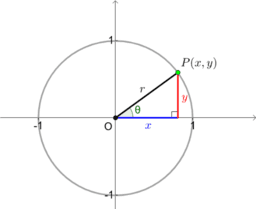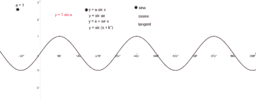# Introduction to trigonometric graphs

A geometric derivation of the basic trigonometric graphs from the unit circle. The unit circle, simply put, is the most basic circle you can get: the circle centred at the origin with a radius of 1. This is a very simple but very powerful mathematical concept, particularly when building up a conceptual understanding of trigonometry. Explore each applet in turn to build up a natural picture of the basic trigonometric graphs (sine, cosine and tangent), before extending into a consideration of transformations. Questions follow each applet to structure your consideration of each graph alongside the unit circle.
•### Trig ratios on the unit circle (SOHCAHTOA)

•### Deriving the sine graph from the unit circle

•### Deriving the cosine graph from the unit circle

•### Deriving the tangent graph from the unit circle

•